output.to from Sideway
Draft for Information Only

# Content

`System of Forces Types of System of Forces`

# System of Forces

In practical applications, there may be more than one force acting on a rigid body or the interested point subjects to the impact of forces acting on the rigid body may be changed. The system of forces may therefore need to be transformed to an equivalent system.The impact of a system of forces acting at a point O on a rigid body can be determined by the vector sum of the forces of the system at point O and the sum of the moment of the forces of the system about the point O.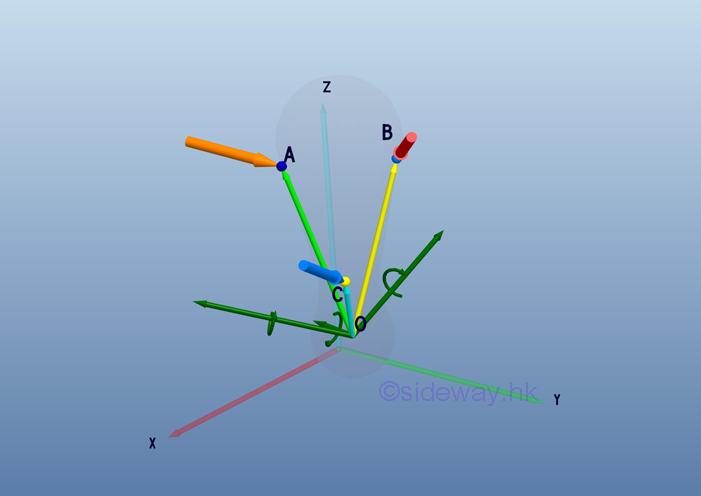Therefore, the equivalent system should includes a force vector R to provide the resultant linear motion and a couple vector MO to provide the resultant rotational motion as the system of forces at point O. When the resultant force vector R is equal to zero, the equivalent system can be reduced to a single couple vector MO system.## Types of System of Forces

In general, forces can be classified according to its point of application and line of action.

Concurrent forces are forces that are applied at the same point. While non concurrent forces are system of forces without a common point of application.Coplanar forces are forces with line of actions that are in the same plane.  When forces with line of actions that are not in a single plane, they are non coplanar forces in space.Parallel  forces are forces with line of actions that are parallel to each other. When system of forces contain force with line of actions are not parallel to others, they are non parallel forces.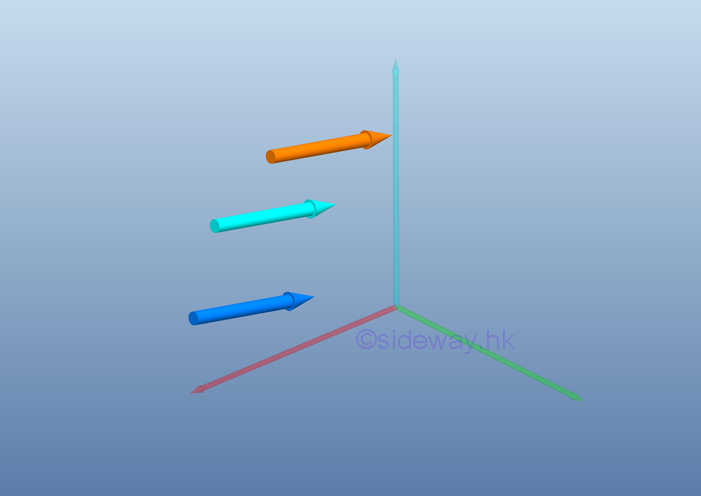And the special case, collinear forces are forces with line of actions that are applied along the same line.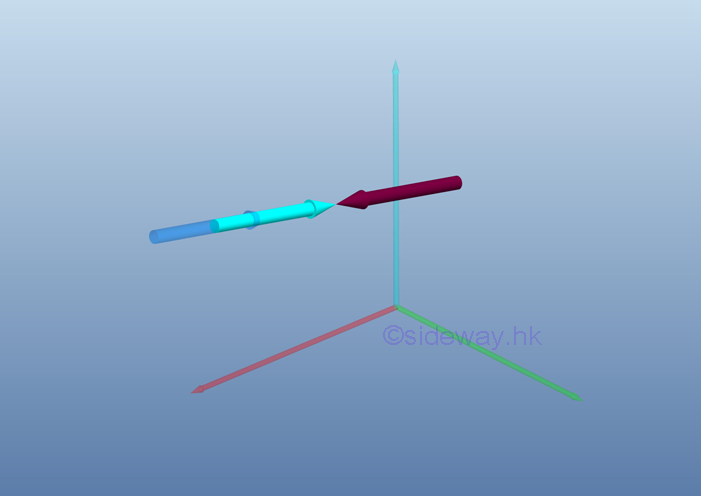Different types of system of forces can be grouped by the combination of classes of forces,

Concurrent forces can be coplanar or non coplanar. Coplanar concurrent  forces system contains forces which met at a single point and lie in a single plane.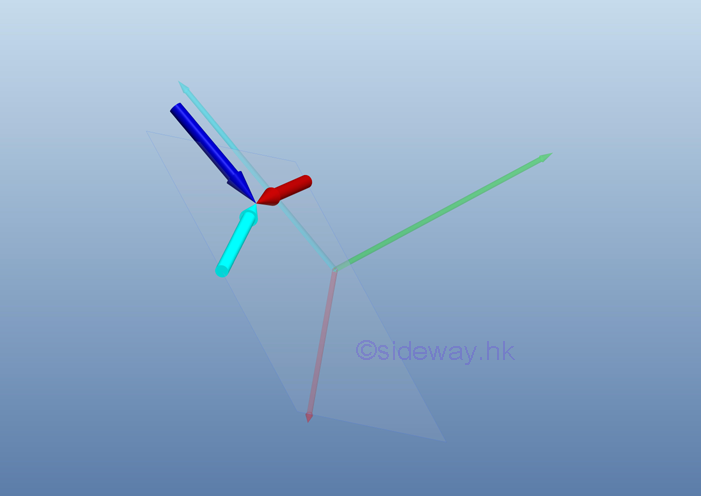While non coplanar concurrent  forces system contains spatial forces which met at a single point but their line of actions do not lie in the same plane.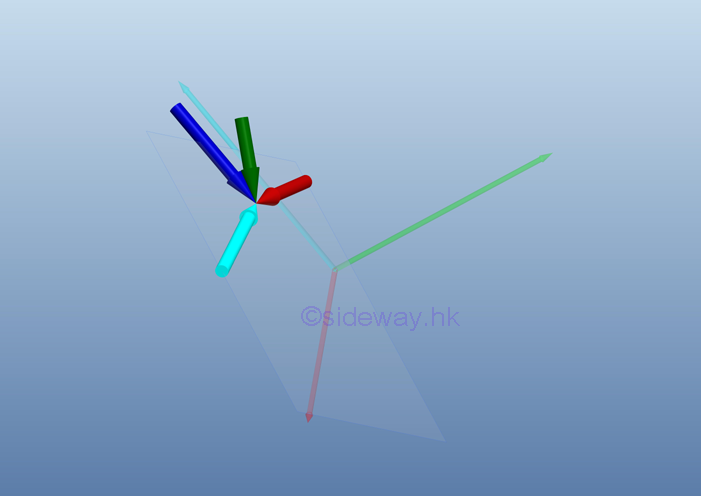Similarly, non concurrent forces can  also be coplanar or non coplanar. Coplanar non concurrent forces system contains forces which do not pass through the same point but their line of actions lie in a single plane.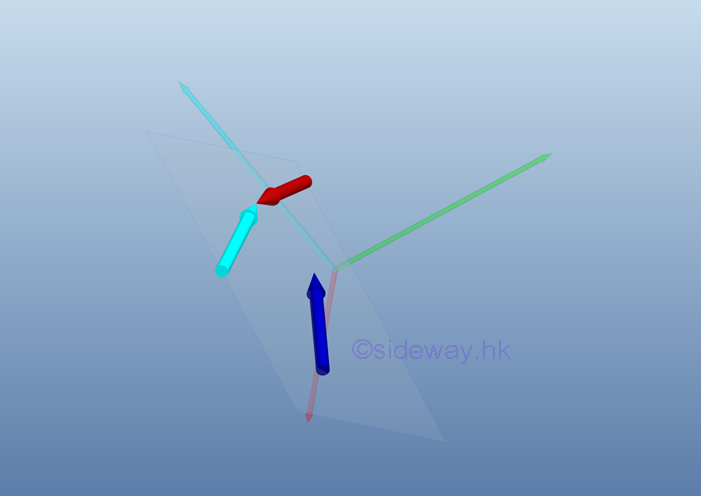While non coplanar non concurrent  forces system contains spatial forces which do not pass through the same point and their line of actions do not lie in the same plane also.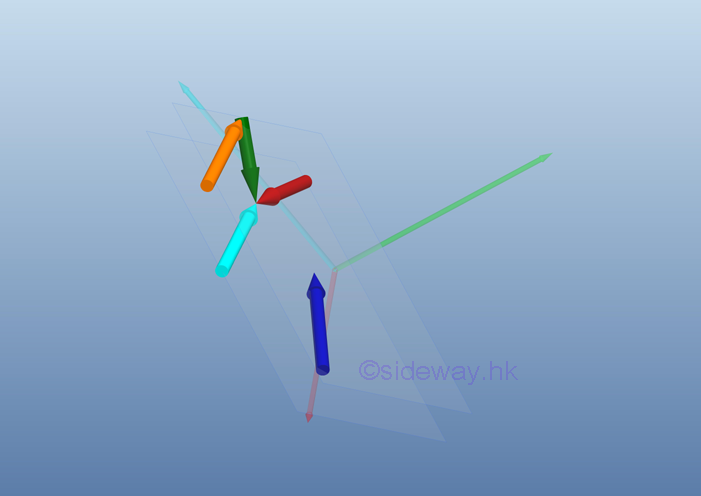Parallel forces are the special case of non concurrent forces. Parallel forces can also be coplanar or non coplanar. Coplanar parallel forces system contains forces with line of actions are parallel to each other and lie in the same plane.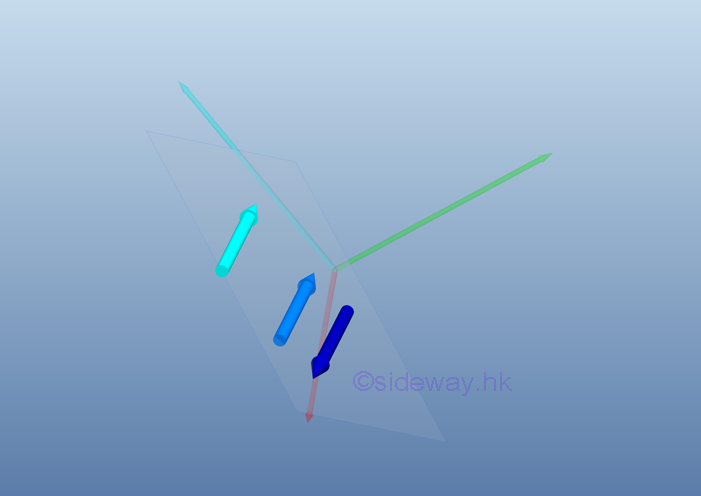While non coplanar parallel forces system contains forces with line of actions are parallel to each other but they are not in the same plane.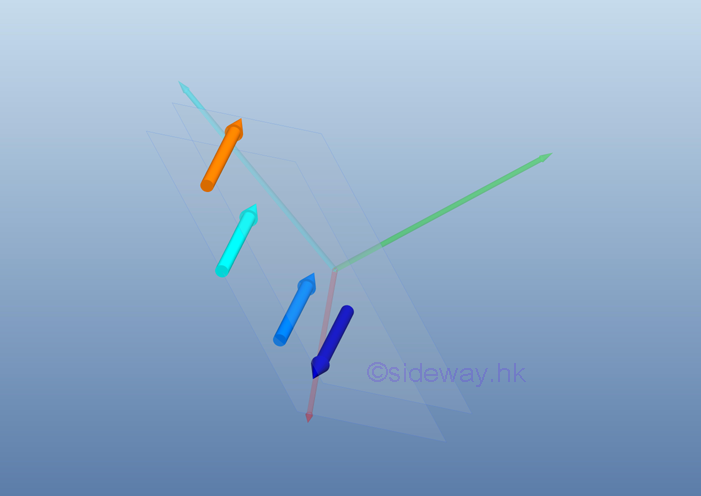ID: 110700013 Last Updated: 8/2/2011 Revision: 1 Ref:References

1. I.C. Jong; B.G. rogers, 1991, Engineering Mechanics: Statics and Dynamics
2. F.P. Beer; E.R. Johnston,Jr.; E.R. Eisenberg, 2004, Vector Mechanics for Engineers: StaticsHome 5

Management

HBR 3

Information

Recreation

Culture

Chinese 1097

English 339

Computer

Hardware 224

Software

Application 213

Latex 52

Manim 204

KB 1

Numeric 19

Programming

Web 289

Unicode 504

HTML 66

CSS 65

SVG 46

ASP.NET 270

OS 429

Python 72

Knowledge

Mathematics

Algebra 84

Geometry 33

Calculus 67

Engineering

Mechanical

Rigid Bodies

Statics 92

Dynamics 37

Control

Natural Sciences

Electric 27